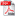# ECUATII DE CALCUL ALE UNOR MARIMI FIZICE ALE SOLUTIEI HIDROAMONIACALE LA SATURATIE

Autor/autori: S.l. ing. Itie-Lica CANDIN, Prof. dr. ing. Constantin lOSIFESCU

Abstract: In this paper, ihere are presented equations for some thermodynamic state parameters of the hydro-ammonia solulion at the saturation point, such as: conceniration-ammonia mass concentraiion 4'=f(T, p) and 4'=f(T, v'), pressure ps= f(T,£'), temperature Ts=f(p,4'). density of the saturated liquid p'=f(T,!;'), and specific volume v'=l/p"= f(T. \'). The equations are presented as polynomial funclions with different number of' coejficienis and for different domains. The results were obtained using different optimisation meihodsfor the coefficients, front the existing numerical data in lables and diagrams.DOWNLOAD PDF